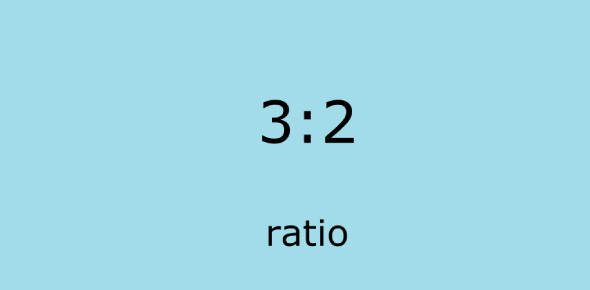# Ratio Test: Math Quiz!

14 Questions | Total Attempts: 726SettingsCreate your own Quiz.

• 1.
Matt sold 35 tickets to the school play and Renee sold 45 tickets. What is the ratio of the number of tickets Matt sold to the number of tickets Renee sold?
• A.

9/7

• B.

7/16

• C.

7/9

• D.

16/7

• 2.
Which ratios are equal to 4: 32?
• A.

2 : 96; 2 : 16

• B.

12 : 96; 2 : 16

• C.

12 : 96; 12 : 16

• D.

2 : 96; 12 : 16

• 3.
The American flag is usually made with its width and length in the ratio of 10 to 19. Which dimensions are in the correct ratio for the flag?
• A.

30 ft by 53 ft

• B.

27 ft by 53 ft

• C.

27 in. by 57 in.

• D.

30 in. by 57 in.

• 4.
Find a ratio equivalent to .
• A.

9/21

• B.

24/9

• C.

15/40

• D.

15/45

• 5.
Write the ratio of 96 runners to 216 swimmers in simplest form.
• A.

96/216

• B.

16/36

• C.

4/9

• D.

9/4

• 6.
Write the ratio of 2 cups to 3 qt as a fraction in simplest form.
• A.

3/2

• B.

2/3

• C.

2/12

• D.

1/6

• 7.
Find a ratio equivalent to 3:25.
• A.

1:9

• B.

9:225

• C.

9:75

• D.

75:9

• 8.
Which fraction shows the ratio of 3 dogs to 5 dogs?
• A.

6/10

• B.

9/10

• C.

27:40

• D.

12:15

• 9.
Find a ratio equivalent to 27/81.
• A.

3:10

• B.

9:9

• C.

1:3

• D.

5:9

• 10.
Maria tossed a coin 20 times and got 12 heads. What is the first step to find the ratio of the number of tails to the total number of tosses?
• A.

Divide 12 by 20

• B.

Subtract 12 from 20

• C.

Multiply 12 by 20

• D.

Add 12 to 20

• 11.
12/24 = 50/100
• A.

True

• B.

False

• 12.
1/3 = 2/9
• A.

True

• B.

False

• 13.
2/3 = 24/36
• A.

True

• B.

False

• 14.
16 to 3 equals 27 to 5.
• A.

True

• B.

False

Related TopicsBack to top
×

Wait!
Here's an interesting quiz for you.